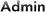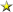#Arx Foris

## Details   Raw Spell Data, Lucy Spell View

 Mana: 0 Casting Time: 2 Recast Time: 0 Fizzle Time: 1.5 Location: Any Time of Day: Any AE Range: 100 Blockable: Yes Focusable: Yes Dispellable: Yes Interruptable: Yes Target Type: Group v1 Spell Type: Beneficial Source: Live 05/20

## Game Description

The power of truth teleports your party into an area inside Sanctus Seru.

## Items with this effect

### Quick Facts

Duration:
Instant
Blocking Arx Foris
Dec 24 2018 at 10:00 PM Rating: Good
Scholar
43 posts
I'm trying to block Arx Foris but it doesn't seem to be blockable. Is there a follow-up effect that this casts that is the actual port spell? I thought I recalled spells saying things like "Teleport q to x, y, z in sseru facing west".
____________________________
Dietrich Conrad <Scions of Time> - Agnarr
Blocking Arx Foris
Dec 29 2018 at 10:08 AM Rating: Excellent5,897 posts
I'm trying to block Arx Foris but it doesn't seem to be blockable. Is there a follow-up effect that this casts that is the actual port spell? I thought I recalled spells saying things like "Teleport q to x, y, z in sseru facing west".

```44515^Tempest Temple Gate^BLUE_TRAIL^tempesttemple^0^0^0^0^7000^1500^10000^0^0^0^150^2522^2112^-1^-1^-1^-1^1^1^1^1^-1^-1^-1^-1^0^1^0^6^25^5^0^24^0^255^255^255^255^255^
255^255^255^255^255^255^100^255^255^255^255^43^0^0^9^0^0^0^0^0^0^0^0^0^0^0^0^0^0^0^0^0^0^100^0^103^252^0^0^0^0^0^0^0^0^0^44515^123^67^0^0^0^0^0^
100^0^0^0^0^0^0^0^0^0^0^100^0^0^0^0^0^0^0^0^50^0^0^0^0^0^0^0^0^0^0^0^1^0^0^1^0^0^-1^0^0^0^22^0^0^1^1^1^-1^0^0^0^8^0^-1^0^1^0^0^1^0^1^0^0^0^0^0^0^
0^1|83|-560|0|100|0\$2|146|-366|0|100|0\$3|146|159|0|100|0\$4|146|38|0|100|0```

Here is the spell code for the wizard spell Tempest Temple Gate. Slot 1 contains the port spell info which is this info in the above, 1|83|-560|0|100|0. This along with the other slot info reports as this in the spell slot info. 1: Teleport to -560,-366,159 in tempesttemple facing NE

Breaking down the first slot SPA info.
1 slot
83 SPA (port)
-560 base1 which is the first coordinate
0 base2
100 calc
0 max

The other slots give the rest of the coordinates but don't show in the spell description because we parse out the info to only show in slot 1.
\$2|146|-366|0|100|0
\$3|146|159|0|100|0
\$4|146|38|0|100|0

Slot 4 has a base1 value of 38 which we just interpret as facing NE.

Now lets look at the raw spell info for Arx Foris.

```2931^Arx Foris^PLAYER_1^^0^100^0^0^2000^1500^0^0^0^0^0^2522^2079^-1^-1^-1^-1^1^1^1^1^-1^-1^-1^-1^0^1^0^3^0^5^0^14^0^255^255^255^255^255^255^255^255^255^
255^255^255^255^255^255^255^44^13^0^40^0^0^0^0^0^0^0^0^0^0^0^0^0^0^0^0^0^0^100^0^116^78^0^0^0^0^0^0^0^0^0^2931^0^0^0^0^0^0^0^100^0^0^0^0^0^0^0^
0^0^0^100^0^0^0^0^0^0^0^0^50^0^0^0^0^0^0^0^0^0^0^0^1^0^0^0^0^0^-1^0^0^0^0^0^0^1^1^1^-1^0^0^0^8^0^-1^0^1^0^0^1^0^1^0^0^0^0^0^0^0^1|10|0|0|100|0```

1|10|0|0|100|0 is the only SPA info this spell reports. SPA 10 is usually just a placeholder spell usually that doesn't report what it does because it casts an ability that's not a spell.

So this is the reason why you don't see coordinates on this spell and probably why you can't block it since it's technically not a spell.
____________________________

To make a Donation directly to me.
https://paypal.me/Gidono
So far I have received 2 donations.
Blocking Arx Foris
Dec 30 2018 at 2:26 AM Rating: Good
Scholar
43 posts
Perfect, thank you for the detailed reply. That makes sense now.
____________________________
Dietrich Conrad <Scions of Time> - Agnarr